# 06：Tensorflow的可视化工具Tensorboard的初步使用

Tensorflow官方推出了可视化工具Tensorboard，可以帮助我们实现以上功能，它可以将模型训练过程中的各种数据汇总起来存在自定义的路径与日志文件中，然后在指定的web端可视化地展现这些信息。

## 1. Tensorboard介绍

### 1.1 Tensorboard的数据形式

Tensorboard可以记录与展示以下数据形式：
（1）标量Scalars
（2）图片Images
（3）音频Audio
（4）计算图Graph
（5）数据分布Distribution
（6）直方图Histograms
（7）嵌入向量Embeddings

### 1.2 Tensorboard的可视化过程

（1）首先肯定是先建立一个graph,你想从这个graph中获取某些数据的信息

（2）确定要在graph中的哪些节点放置summary operations以记录信息

….

（3）operations并不会去真的执行计算，除非你告诉他们需要去run,或者它被其他的需要run的operation所依赖。而我们上一步创建的这些summary operations其实并不被其他节点依赖，因此，我们需要特地去运行所有的summary节点。但是呢，一份程序下来可能有超多这样的summary 节点，要手动一个一个去启动自然是及其繁琐的，因此我们可以使用tf.summary.merge_all去将所有summary节点合并成一个节点，只要运行这个节点，就能产生所有我们之前设置的summary data。

（4）使用tf.summary.FileWriter将运行后输出的数据都保存到本地磁盘中

（5）运行整个程序，并在命令行输入运行tensorboard的指令，之后打开web端可查看可视化的结果

## 2.Tensorboard使用案例

### 2.1 导入包，定义超参数，载入数据

（1）首先还是导入需要的包：

from __future__ import absolute_import
from __future__ import division
from __future__ import print_function

import argparse
import sys

import tensorflow as tf

from tensorflow.examples.tutorials.mnist import input_data

（2）定义固定的超参数,方便待使用时直接传入。如果你问，这个超参数为啥要这样设定，如何选择最优的超参数？这个问题此处先不讨论，超参数的选择在机器学习建模中最常用的方法就是“交叉验证法”。而现在假设我们已经获得了最优的超参数，设置学利率为0.001，dropout的保留节点比例为0.9，最大循环次数为1000.

max_step = 1000  # 最大迭代次数
learning_rate = 0.001   # 学习率
dropout = 0.9   # dropout时随机保留神经元的比例

data_dir = ''   # 样本数据存储的路径
log_dir = ''    # 输出日志保存的路径

（3）接着加载数据,下载数据是直接调用了tensorflow提供的函数read_data_sets,输入两个参数，第一个是下载到数据存储的路径，第二个one_hot表示是否要将类别标签进行独热编码。它首先回去找制定目录下有没有这个数据文件，没有的话才去下载，有的话就直接读取。所以第一次执行这个命令，速度会比较慢。

mnist = input_data.read_data_sets(data_dir,one_hot=True)

### 2.2 创建特征与标签的占位符，保存输入的图片数据到summary

（1）创建tensorflow的默认会话：

sess = tf.InteractiveSession()

（2）创建输入数据的占位符，分别创建特征数据x，标签数据y_

with tf.name_scope('input'):
x = tf.placeholder(tf.float32, [None, 784], name='x-input')
y_ = tf.placeholder(tf.float32, [None, 10], name='y-input')

（3）使用tf.summary.image保存图像信息

tf.summary.image（）中传入的第一个参数是命名，第二个是图片数据，第三个是最多展示的张数，此处为10张

with tf.name_scope('input_reshape'):
image_shaped_input = tf.reshape(x, [-1, 28, 28, 1])
tf.summary.image('input', image_shaped_input, 10)

### 2.3 创建初始化参数的方法，与参数信息汇总到summary的方法

（1）在构建神经网络模型中，每一层中都需要去初始化参数w,b,为了使代码简介美观，最好将初始化参数的过程封装成方法function。

def weight_variable(shape):
"""Create a weight variable with appropriate initialization."""
initial = tf.truncated_normal(shape, stddev=0.1)
return tf.Variable(initial)

def bias_variable(shape):
"""Create a bias variable with appropriate initialization."""
initial = tf.constant(0.1, shape=shape)
return tf.Variable(initial)

（2）我们知道，在训练的过程在参数是不断地在改变和优化的，我们往往想知道每次迭代后参数都做了哪些变化，可以将参数的信息展现在tenorbord上，因此我们专门写一个方法来收录每次的参数信息。

def variable_summaries(var):
"""Attach a lot of summaries to a Tensor (for TensorBoard visualization)."""
with tf.name_scope('summaries'):
# 计算参数的均值，并使用tf.summary.scaler记录
mean = tf.reduce_mean(var)
tf.summary.scalar('mean', mean)

# 计算参数的标准差
with tf.name_scope('stddev'):
stddev = tf.sqrt(tf.reduce_mean(tf.square(var - mean)))
# 使用tf.summary.scaler记录记录下标准差，最大值，最小值
tf.summary.scalar('stddev', stddev)
tf.summary.scalar('max', tf.reduce_max(var))
tf.summary.scalar('min', tf.reduce_min(var))
# 用直方图记录参数的分布
tf.summary.histogram('histogram', var)

### 2.4 构建神经网络层

（1）创建第一层隐藏层

input_tensor：特征数据
input_dim：输入数据的维度大小
output_dim：输出数据的维度大小(=隐层神经元个数）
layer_name：命名空间
act=tf.nn.relu：激活函数（默认是relu)

def nn_layer(input_tensor, input_dim, output_dim, layer_name, act=tf.nn.relu):
"""Reusable code for making a simple neural net layer.
It does a matrix multiply, bias add, and then uses relu to nonlinearize.
It also sets up name scoping so that the resultant graph is easy to read,
and adds a number of summary ops.
"""
# 设置命名空间
with tf.name_scope(layer_name):
# 调用之前的方法初始化权重w，并且调用参数信息的记录方法，记录w的信息
with tf.name_scope('weights'):
weights = weight_variable([input_dim, output_dim])
variable_summaries(weights)
# 调用之前的方法初始化权重b，并且调用参数信息的记录方法，记录b的信息
with tf.name_scope('biases'):
biases = bias_variable([output_dim])
variable_summaries(biases)
# 执行wx+b的线性计算，并且用直方图记录下来
with tf.name_scope('linear_compute'):
preactivate = tf.matmul(input_tensor, weights) + biases
tf.summary.histogram('linear', preactivate)
# 将线性输出经过激励函数，并将输出也用直方图记录下来
activations = act(preactivate, name='activation')
tf.summary.histogram('activations', activations)

# 返回激励层的最终输出
return activations

hidden1 = nn_layer(x, 784, 500, 'layer1')

（2）创建一个dropout层，,随机关闭掉hidden1的一些神经元，并记录keep_prob

 with tf.name_scope('dropout'):
keep_prob = tf.placeholder(tf.float32)
tf.summary.scalar('dropout_keep_probability', keep_prob)
dropped = tf.nn.dropout(hidden1, keep_prob)

（3）创建一个输出层，输入的维度是上一层的输出:500,输出的维度是分类的类别种类：10，激活函数设置为全等映射identity.（暂且先别使用softmax,会放在之后的损失函数中一起计算）

y = nn_layer(dropped, 500, 10, 'layer2', act=tf.identity)

### 2.5 创建损失函数

with tf.name_scope('loss'):
# 计算交叉熵损失（每个样本都会有一个损失）
diff = tf.nn.softmax_cross_entropy_with_logits(labels=y_, logits=y)
with tf.name_scope('total'):
# 计算所有样本交叉熵损失的均值
cross_entropy = tf.reduce_mean(diff)

tf.summary.scalar('loss', cross_entropy)

### 2.6 训练，并计算准确率

（1）使用AdamOptimizer优化器训练模型，最小化交叉熵损失

with tf.name_scope('train'):
train_step = tf.train.AdamOptimizer(learning_rate).minimize(
cross_entropy)

（2）计算准确率,并用tf.summary.scalar记录准确率

with tf.name_scope('accuracy'):
with tf.name_scope('correct_prediction'):
# 分别将预测和真实的标签中取出最大值的索引，弱相同则返回1(true),不同则返回0(false)
correct_prediction = tf.equal(tf.argmax(y, 1), tf.argmax(y_, 1))
with tf.name_scope('accuracy'):
# 求均值即为准确率
accuracy = tf.reduce_mean(tf.cast(correct_prediction, tf.float32))
tf.summary.scalar('accuracy', accuracy)

### 2.7 合并summary operation, 运行初始化变量

# summaries合并
merged = tf.summary.merge_all()
# 写到指定的磁盘路径中
train_writer = tf.summary.FileWriter(log_dir + '/train', sess.graph)
test_writer = tf.summary.FileWriter(log_dir + '/test')

# 运行初始化所有变量
tf.global_variables_initializer().run()

### 2.8 准备训练与测试的两个数据，循环执行整个graph进行训练与评估

（1）现在我们要获取之后要喂人的数据.

def feed_dict(train):
"""Make a TensorFlow feed_dict: maps data onto Tensor placeholders."""
if train:
xs, ys = mnist.train.next_batch(100)
k = dropout
else:
xs, ys = mnist.test.images, mnist.test.labels
k = 1.0
return {x: xs, y_: ys, keep_prob: k}

（2）开始训练模型。

for i in range(max_steps):
if i % 10 == 0:  # 记录测试集的summary与accuracy
summary, acc = sess.run([merged, accuracy], feed_dict=feed_dict(False))
test_writer.add_summary(summary, i)
print('Accuracy at step %s: %s' % (i, acc))
else:  # 记录训练集的summary
if i % 100 == 99:  # Record execution stats
run_options = tf.RunOptions(trace_level=tf.RunOptions.FULL_TRACE)
run_metadata = tf.RunMetadata()
summary, _ = sess.run([merged, train_step],
feed_dict=feed_dict(True),
options=run_options,
run_metadata=run_metadata)
train_writer.add_run_metadata(run_metadata, 'step%03d' % i)
train_writer.add_summary(summary, i)
print('Adding run metadata for', i)
else:  # Record a summary
summary, _ = sess.run([merged, train_step], feed_dict=feed_dict(True))
train_writer.add_summary(summary, i)
train_writer.close()
test_writer.close()

### 2.9 执行程序，tensorboard生成可视化

（1）运行整个程序，在程序中定义的summary node就会将要记录的信息全部保存在指定的logdir路径中了，训练的记录会存一份文件，测试的记录会存一份文件。

（2）进入linux命令行，运行以下代码，等号后面加上summary日志保存的路径（在程序第一步中就事先自定义了）

tensorboard --logdir=

Starting TensorBoard 41 on port 6006
(You can navigate to http://127.0.1.1:6006)


http://127.0.1.1:6006在浏览器中打开，成功的话如下：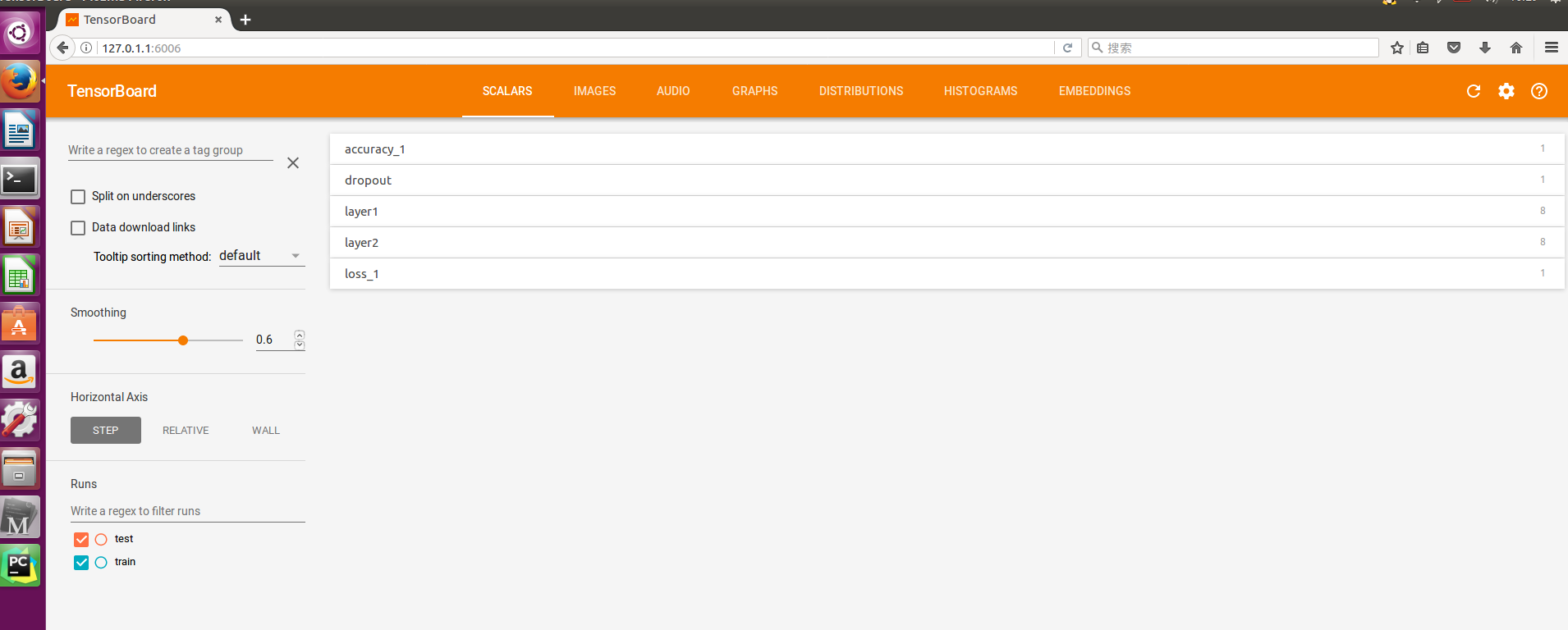### 2.10 Tensorboard Web端解释

（1）SCALARS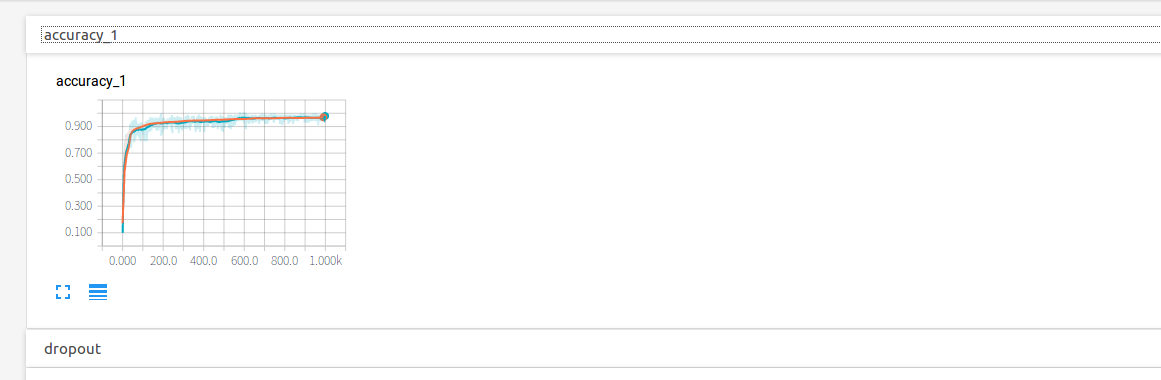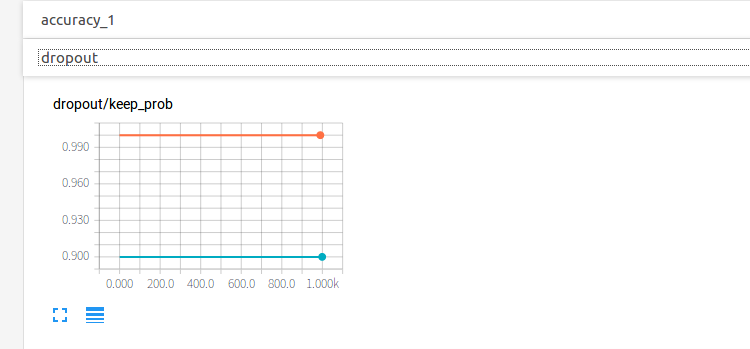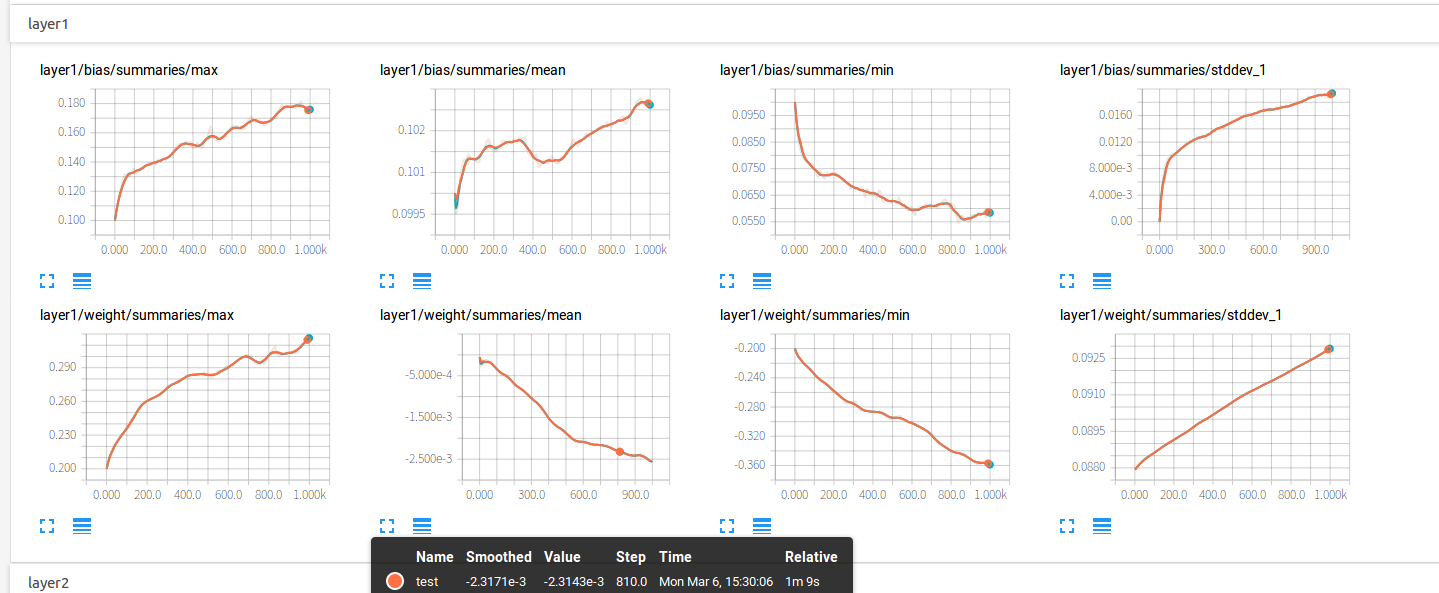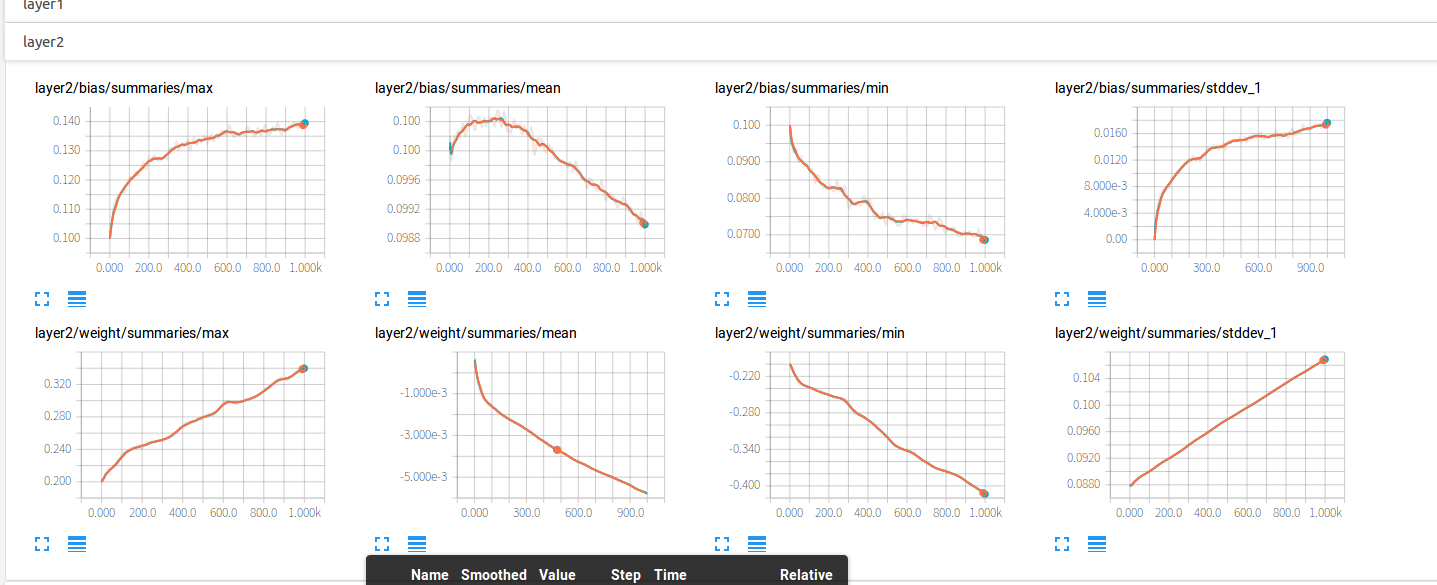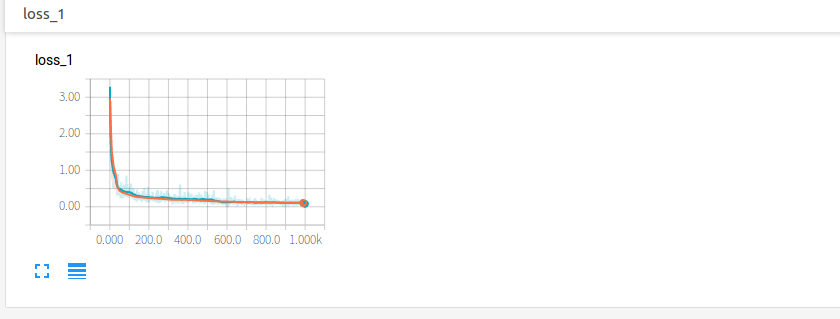（2）IMAGES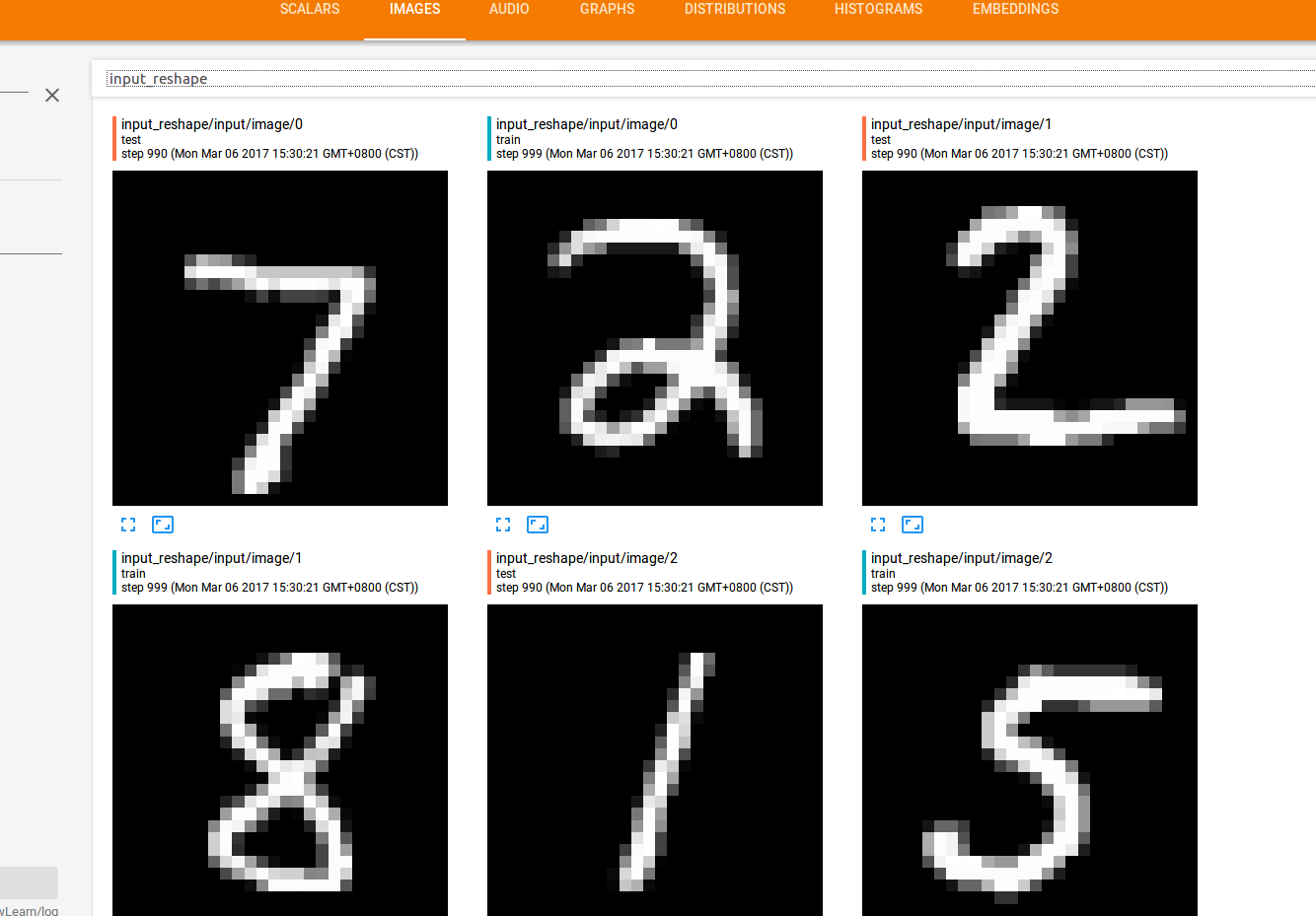（3）AUDIO

（4）GRAPHS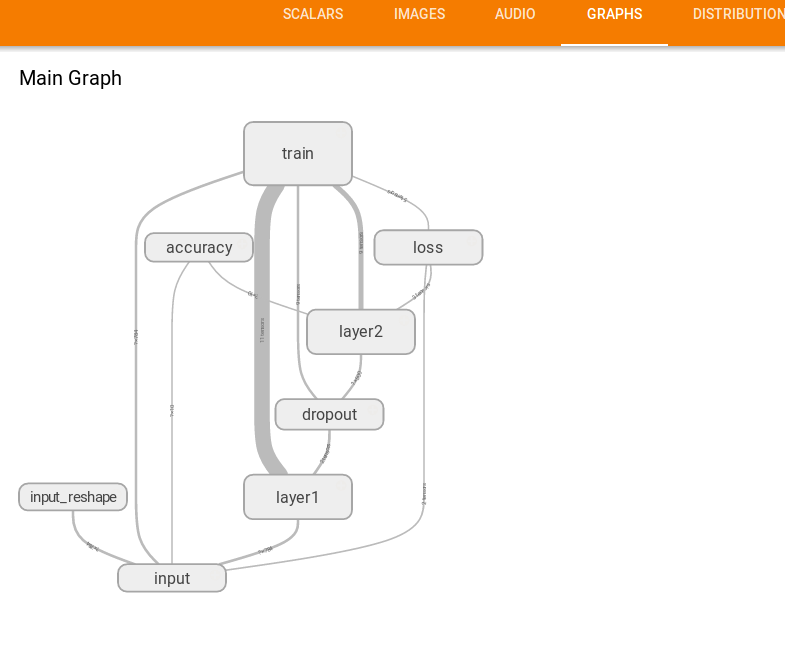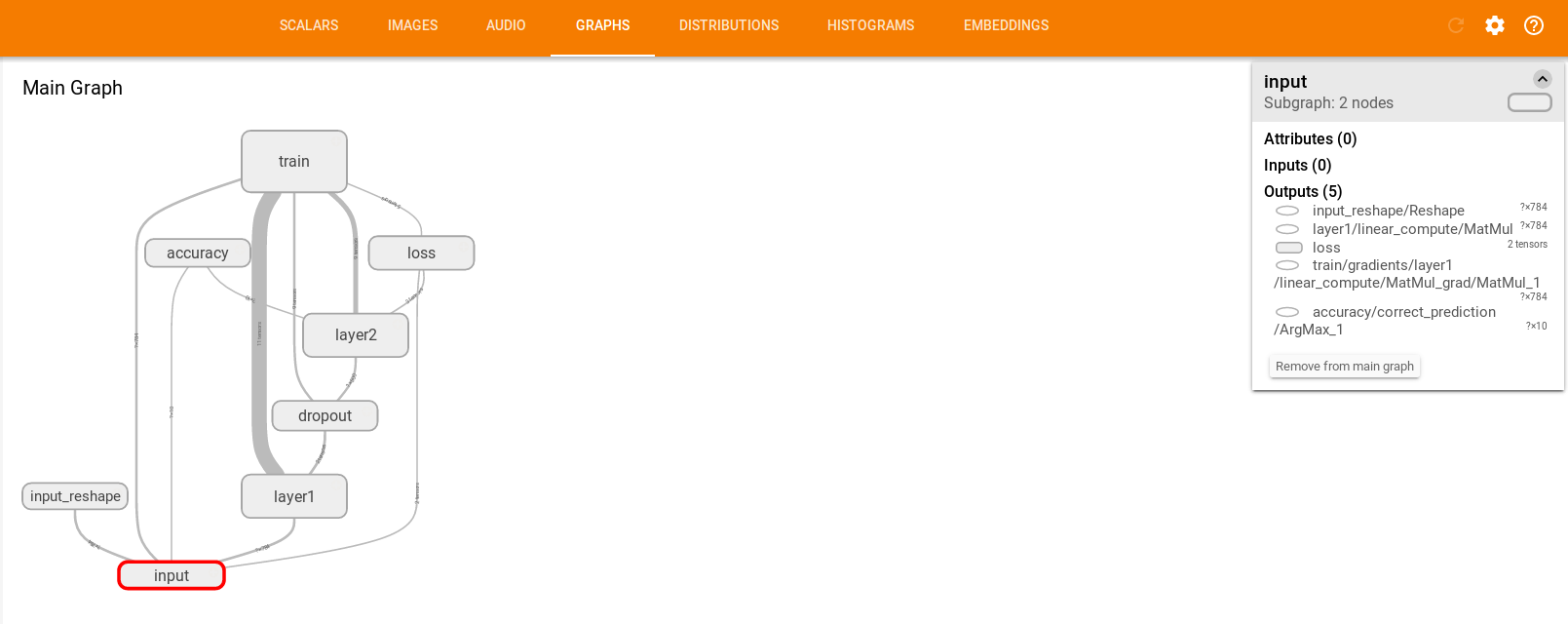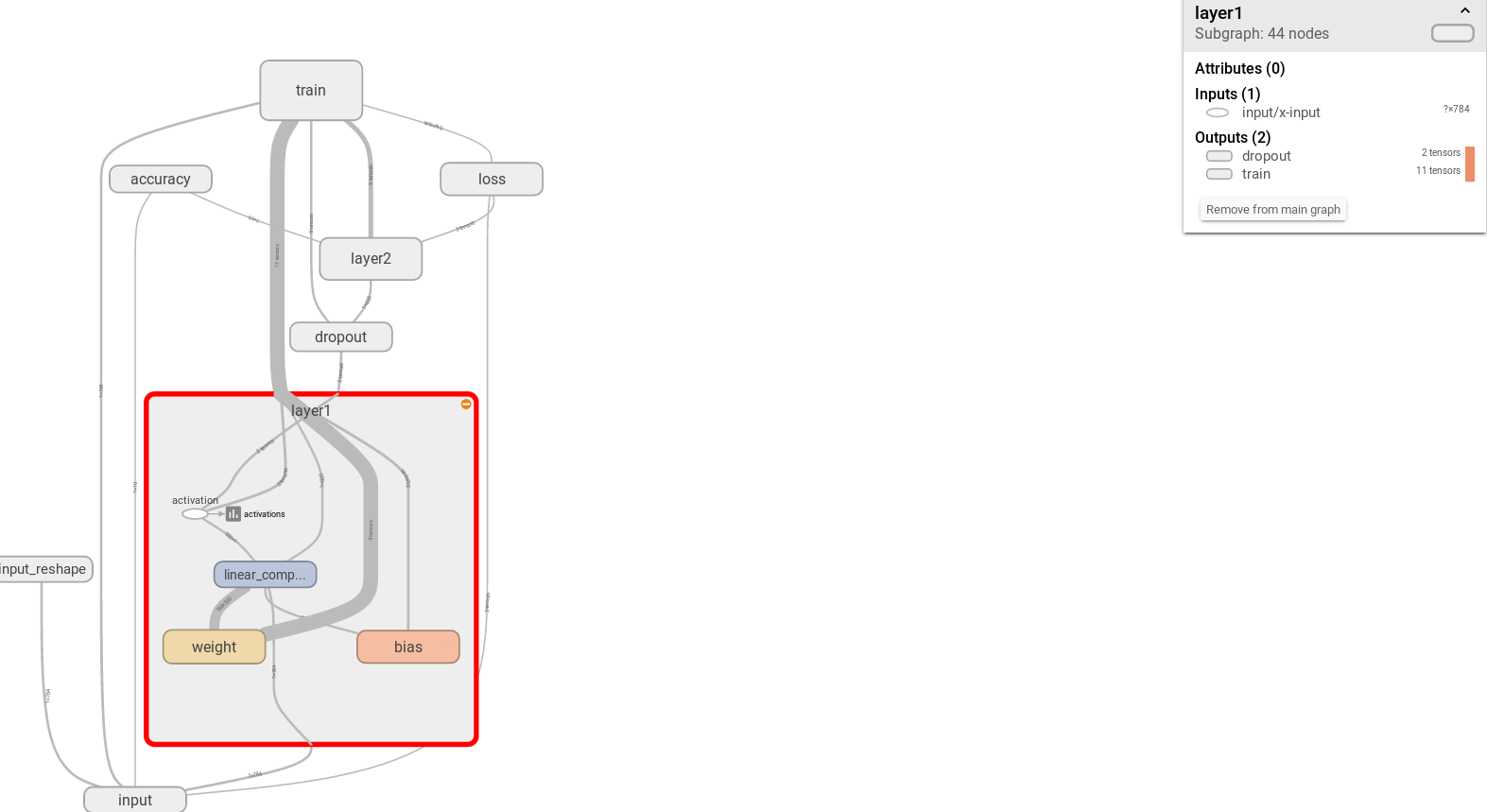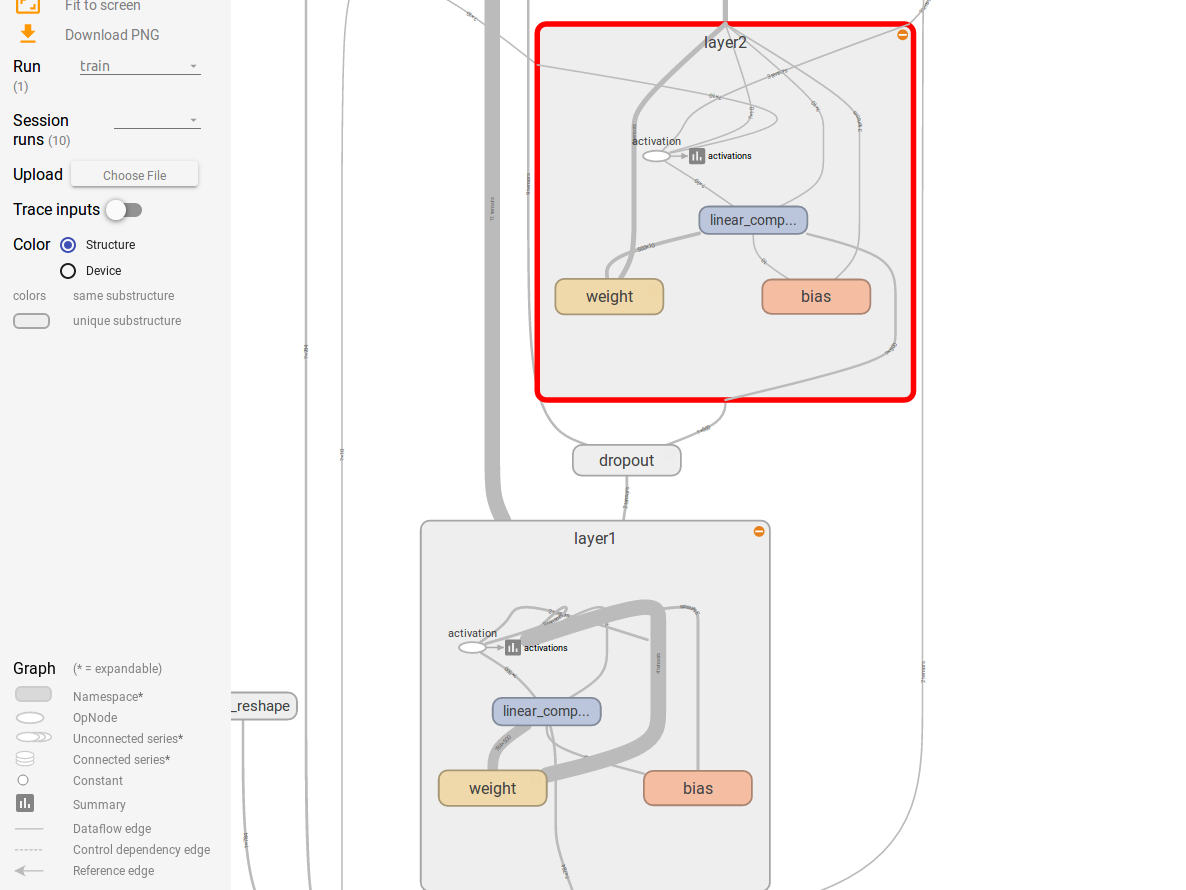（5）DISTRIBUTIONS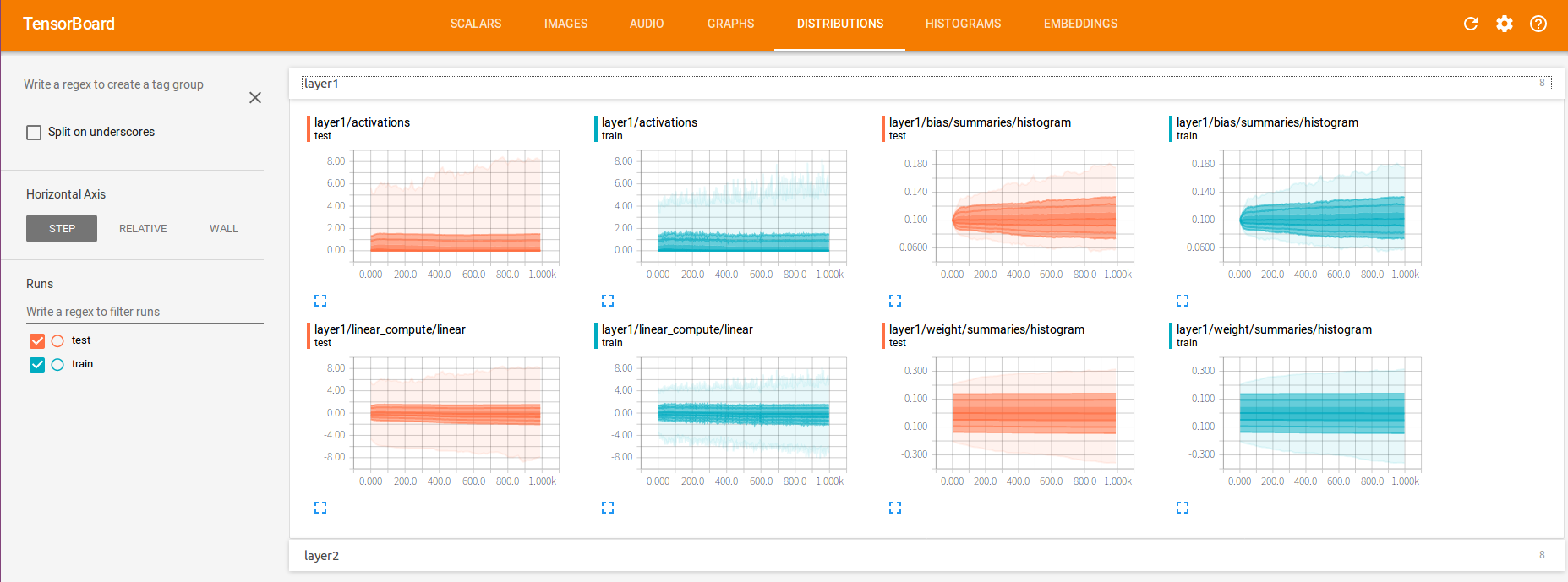（6）HISTOGRAMS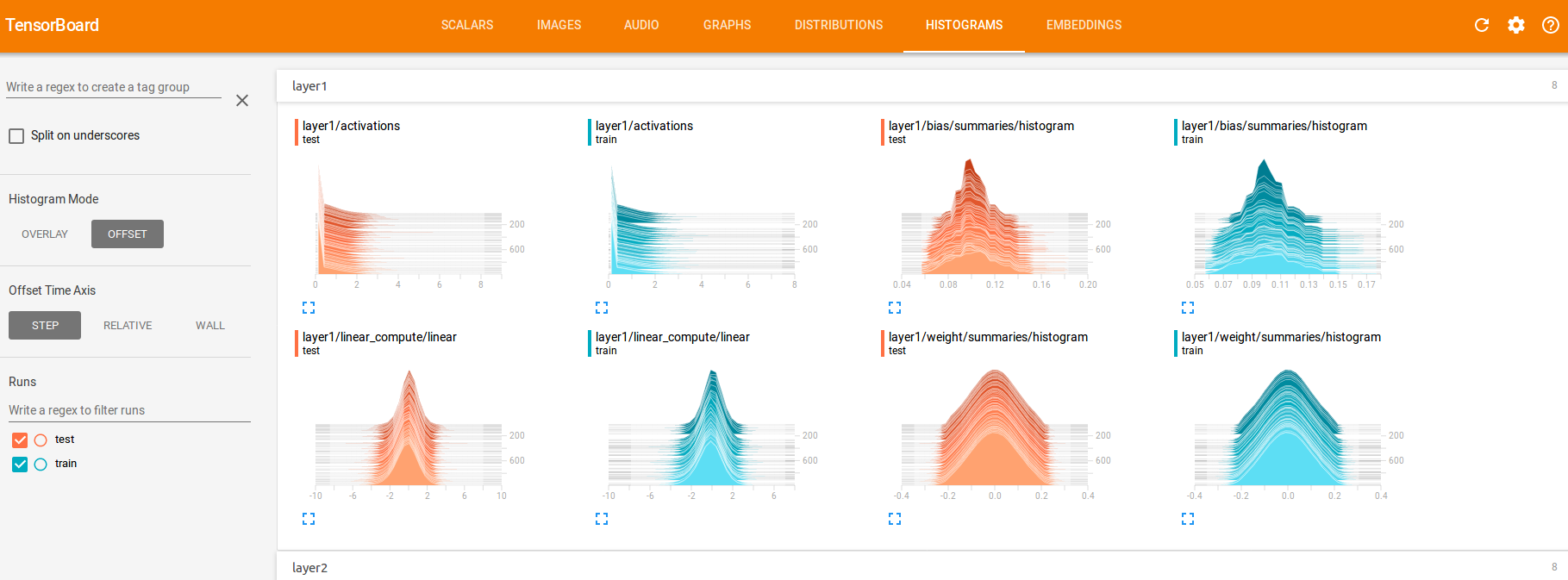（7）EMBEDDINGS02-287万+
11-051万+
08-069万+
05-151万+
02-208万+
08-19337
09-261万+
05-198143
05-161万+
04-116万+
11-232万+
03-111500
08-19679
07-065万+
11-223万+
07-192万+
02-275万+
©️2020 CSDN 皮肤主题: 终极编程指南 设计师:CSDN官方博客